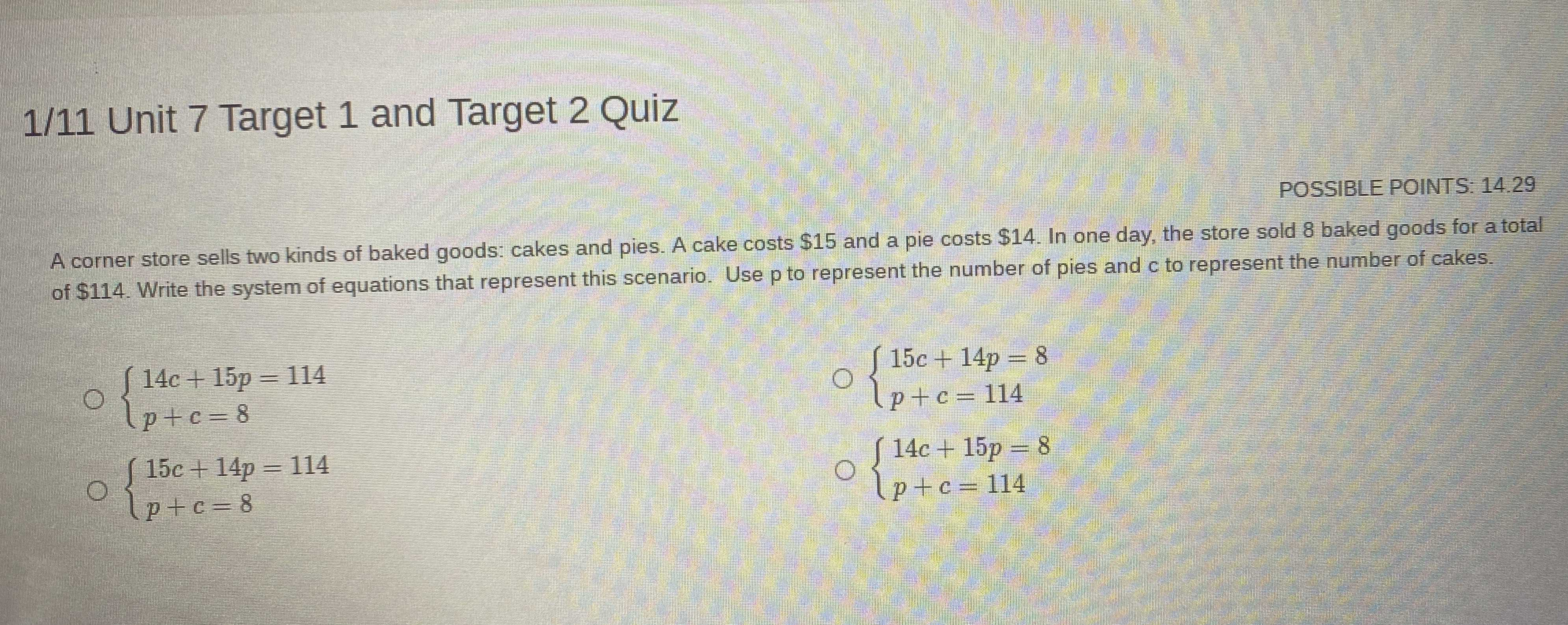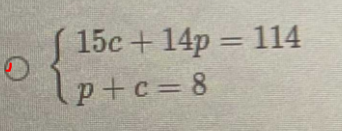### ¿Todavía tienes preguntas de matemáticas?

Pregunte a nuestros tutores expertos
Algebra
PreguntaA corner store sells two kinds of baked goods: cakes and pies. A cake costs $$\ 15$$ and a pie costs $$\ 14$$ . In one day, the store sold $$8$$ baked goods for a total of $$\ 114$$ . Write the system of equations that represent this scenario. Use $$p$$ to represent the number of pies and $$c$$ to represent the number of cakes.